Courses

# Test: Introduction To Quadratic Equations

## 30 Questions MCQ Test Mathematics (Maths) Class 10 | Test: Introduction To Quadratic Equations

Description
This mock test of Test: Introduction To Quadratic Equations for Class 10 helps you for every Class 10 entrance exam. This contains 30 Multiple Choice Questions for Class 10 Test: Introduction To Quadratic Equations (mcq) to study with solutions a complete question bank. The solved questions answers in this Test: Introduction To Quadratic Equations quiz give you a good mix of easy questions and tough questions. Class 10 students definitely take this Test: Introduction To Quadratic Equations exercise for a better result in the exam. You can find other Test: Introduction To Quadratic Equations extra questions, long questions & short questions for Class 10 on EduRev as well by searching above.
QUESTION: 1

### Write the general form of a quadratic polynomia

Solution:

If the coefficient of x2 is zero , then the equation is not a quadratic equation , its a linear equation. So its necessary condition for the quadratic equation.

QUESTION: 2

### One of the roots of the quadratic equation 6x2 – x – 2 = 0 is:​

Solution:
*Multiple options can be correct
QUESTION: 3

### Which of the following statement is TRUE?

Solution:

A quadratic equation in variable x is of the form ax2+ bx + c = 0, where a, b, c are real numbers a ≠ o, because if a=0 then the equation becomes a linear equation.
If we can factorise ax2 + bx + c, a ≠ 0 into product of two linear factors then roots can be found by equating each factor to zero because if two factors are in multiplication and equal to zero then either of the factor is zero.
A real number R is said to be a root of the quadratic equation ax2 + bx + c = 0 if a(R)2 + bR + c = 0. , root means that the value gives answer equal to zero.
So all are correct.

QUESTION: 4

If n is a non negative integer, then ann +…+ a 1 x + a0 is a

Solution:
QUESTION: 5

The two positive numbers differ by 5 and square of their sum is 169 are

Solution:
QUESTION: 6

If 4 is a root of the equation x2 + 3x + k = 0, then k is​

Solution:

4 is the solution , this means that if we put x=4 we get 0. So putting x=4 in the equation x2+3x+k=0 we get 42+3*4+k=0
16+12+k=0 ⇒ k=-28

QUESTION: 7

If 8 is a root of the equation x2 – 10x + k = 0, then the value of k is:​

Solution:

Let, p(x) = x²-10x+k
since, 8 is the root of p(x)
∴ p(8) = 0
8²-10(8)+k = 0
64-80+k = 0
-16+k = 0
⇒ k = 16 .

QUESTION: 8

Which of following is not a quadratic equation:

Solution:
QUESTION: 9

The solution of 5z2 = 3z is​

Solution:

We have 5z2=3z
5z2-3z=0
z(5z-3)=0
So either z=0
Or 5z-3 =0  = z=⅗. So there are two solutions

QUESTION: 10

Which of the following equations has 2 as a root?

Solution:
QUESTION: 11

Which of the following equations has the sum of its roots as 3?

Solution:
*Multiple options can be correct
QUESTION: 12

Which of the following is not a quadratic equation:​

Solution:

Option (B) and (D) , both are the correct answers.  We have x(x + 1) + 8 = (x + 2) (x – 2)
=x+ x + 8 = x2 - 4
= x = -12, which is not a quadratic equation
Also, in (B) (x + 2)2 = x3 – 4
=x+4x + 4=x- 4, which is a cubic equation

QUESTION: 13

If x = -2 is a root of equation x2 – 4x + K = 0 then value of K is

Solution:
QUESTION: 14

The same value of x satisfies the equations 4x + 5 = 0 and 4x2 + (5 + 3p)x + 3p= 0, then p is​

Solution:
QUESTION: 15

If x = 1 is a root of equation x2 – Kx + 5 = 0 then value of K is

Solution:

Root of the equation means that the value when substituted in the equation gives zero as answer.
x- kx + 5 = 0
Putting x = 1
1*1 -k + 5 = 0
-k+6=0
k=6

QUESTION: 16

The equation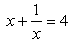in standard form ax2 + bx + c = 0 is written as :

Solution:

We have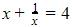Taking LCM,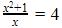Multiplying LHS and RHS by x
x2+1=4x
x2-4x+1=0
Which is the required equation.

QUESTION: 17

If x = 1 is a common root of the equation x2 + ax – 3 = 0 and bx2 – 7x + 2 = 0 then ab =

Solution:
QUESTION: 18

If the area of a rectangle is 24 m2 and its perimeter is 20 m, the equation to find its length and breadth would be:​

Solution:
QUESTION: 19

If x = 2 is a root of equation x2 + 3x – k = 0 then value of k is

Solution:
QUESTION: 20

The roots of the equation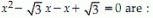Solution:

x- √3x - x + √3 = 0
Taking common x from first two terms
x (x - √3) - 1 (x - √3) = 0
(x - √3) (x - 1) = 0
So, either x = √3 or x = 1

QUESTION: 21

The condition for equation ax2 + bx + c = 0 to be quadratic is​

Solution:

For ax+ bx + c = 0 to be a quadratic equation a0because if a is zero then ax= 0 So we are left with only bx + c = 0 which is a linear equation in one variable. So to be a quadratic equation ax2 cannot be zero.

QUESTION: 22

The value of q if x = 2 is a solution of 8x2 + qx – 4 = 0 is _____​

Solution:
QUESTION: 23

The equation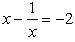in standard form ax2 + bx + c = 0 is written as

Solution:
QUESTION: 24

Zeroes of the quadratic polynomial ax2 + bx + c and roots of the quadratic equation ax2 + bx + c = 0 are ——–​

Solution:

Zeros of the polynomial means the value of variable such that the equation is equal to zero.Roots of the equation means the value of the variable for which LHS=RHS which basically means that the equation is equal to zero. Hence Zeros and roots are one and the same thing.

QUESTION: 25

The condition for equation ax2 + bx + c = 0 to be linear is​

Solution:
QUESTION: 26

The solution of x2 + 4x + 4 = 0 is​

Solution:
QUESTION: 27

If x2 + 2 kx + 4 = 0 has a root x = 2, then the value of k is?

Solution:
QUESTION: 28

Solve 9x2 = 36​

Solution:
QUESTION: 29

The value/s of x when (x – 4) (3x + 2) = 0 ________​

Solution:
QUESTION: 30

The positive root of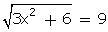is

Solution: